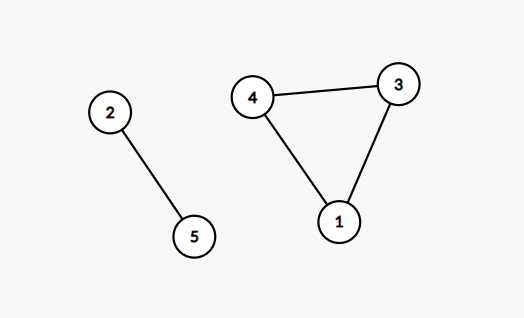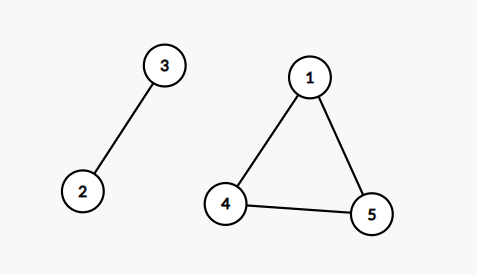## Graph Theory: Connectivity

Points: 10
Time limit: 2.0s
Memory limit: 128M

Problem type

Given an undirected graph with nodes labelled from $$1$$ to $$N$$, check whether or not node $$N$$ can be reached from node $$1$$.

#### Input Specification

The first line of the input will contain two integers $$N$$ and $$M$$ $$(1 \le N, M \le 10^5)$$, indicating the number of nodes and edges.

The next $$M$$ lines will each contain two integers $$a_i$$ and $$b_i$$ $$(1 \le a_i, b_i \le N)$$, representing a bidirectional edge connecting the nodes $$a_i$$ and $$b_i$$.

#### Output Specification

Output YES if node $$N$$ can be reached and NO otherwise.

#### Sample Input 1

5 4
1 4
4 3
3 1
2 5

#### Sample Output 1

NO

#### Sample Explanation 1

The following image shows the graph found in the sample input.#### Sample Input 2

5 4
1 4
5 4
1 5
2 3

#### Sample Output 2

YES

#### Sample Explanation 2

The following image shows the graph found in the sample input. There are $$2$$ possible paths from $$1$$:

• 1 - 4 - 5
• 1 - 5